Race Results 1997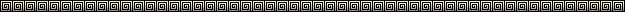WSMC OVERALL ~ 19TH Place
500 Singles ~ 2nd Place
Formula Singles ~ 6th Place
Vintage Dinosaurs ~ 7th Place
Toyota Gold Cup Unlimited Formula 1 ~ ???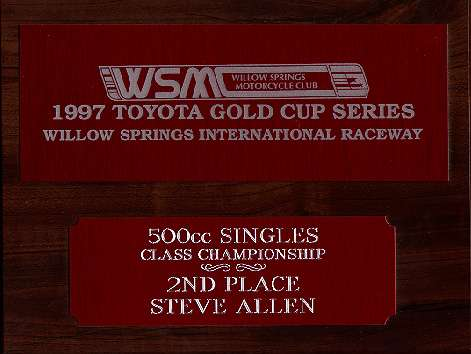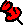Race classes will be abbreviated in the following manner:
FS = Formula Singles
500 = 500 Singles
V = Vintage Dinosaurs
SS = 750 Super Stock
MP = 750 Modified Production
SB = 750 Super Bike
F1 = Formula 1
SOS-F1 = Sound of Singles Formula 1
SOS-F2 = Sound of Singles Formula 2
O/A = Over AllWSMC rnd 1:  500=3rd, FS=DNF, V=DNS  (motor went away)
WSMC rnd 2:  missed due to illness
WSMC rnd 3:  500=3rd, FS=8th,V=6th, SB=16th, O/A=49th
WSMC rnd 4:  500=3rd, FS=5th, V=6th, F1=14th, O/A=29th
AHRMA (april @ WSIR ~ saturday) ~ SOS-F1=13th, SOS-F2=4th
AHRMA (april @ WSIR ~ sunday) ~ SOS-F1=14th, SOS-F2=3rd
WSMC rnd 5:  500=2nd, FS=5th, V=6th, F1=13th, O/A=26th
WSMC rnd 6:  500=2nd, FS=6th, V=6th, F1=18th, O/A= 22th
WSMC rnd 7: 500=1st, FS=8th, V=11th, F1=21st, O/A=20TH
WSMC rnd 8: 500=2ND, FS=6TH, V=7TH, F1=8TH, O/A=21st
AHRMA (8/23 ~ Sears Pt) ~ SOS F1=13th, SOS F2=6th
AHRMA (8/24 ~ Sears Pt) ~ SOS F1=11th, SOS F2=6th
WSMC rnd 9: 500=2nd, FS=5th, V=9th, F1=16th, O/A=20th
WSMC rnd 10: 500=2nd, FS=8th, V=6th, F1=15th, O/A=19th
WSMC rnd 11: V=DNF, F1=19th, O/A=21st (crash in vintage race)
WSMC rnd 12: 500=2nd, FS=4th, V=7th, SB=7th, F1=15th, O/A=19th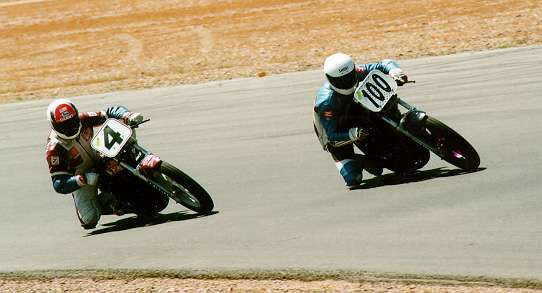Arch rival and good friend Danny Farnsworth and I in the heat of battle in T2 at Willow Springs International Raceway during the 500 Singles race, Round 10, 1997.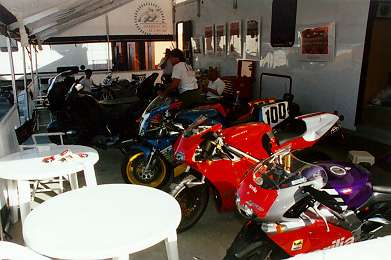Slumin' in The Silverman Museum Racing pit at Willow Springs. Note Jack Silverman's Aprilia 250, Ducati 996 & Ducati Supermono along with Vicky Jackson-Bell's 125 off in the background...... My GSX-R felt right at home!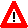All images, pictures & information contained within this website
property of &